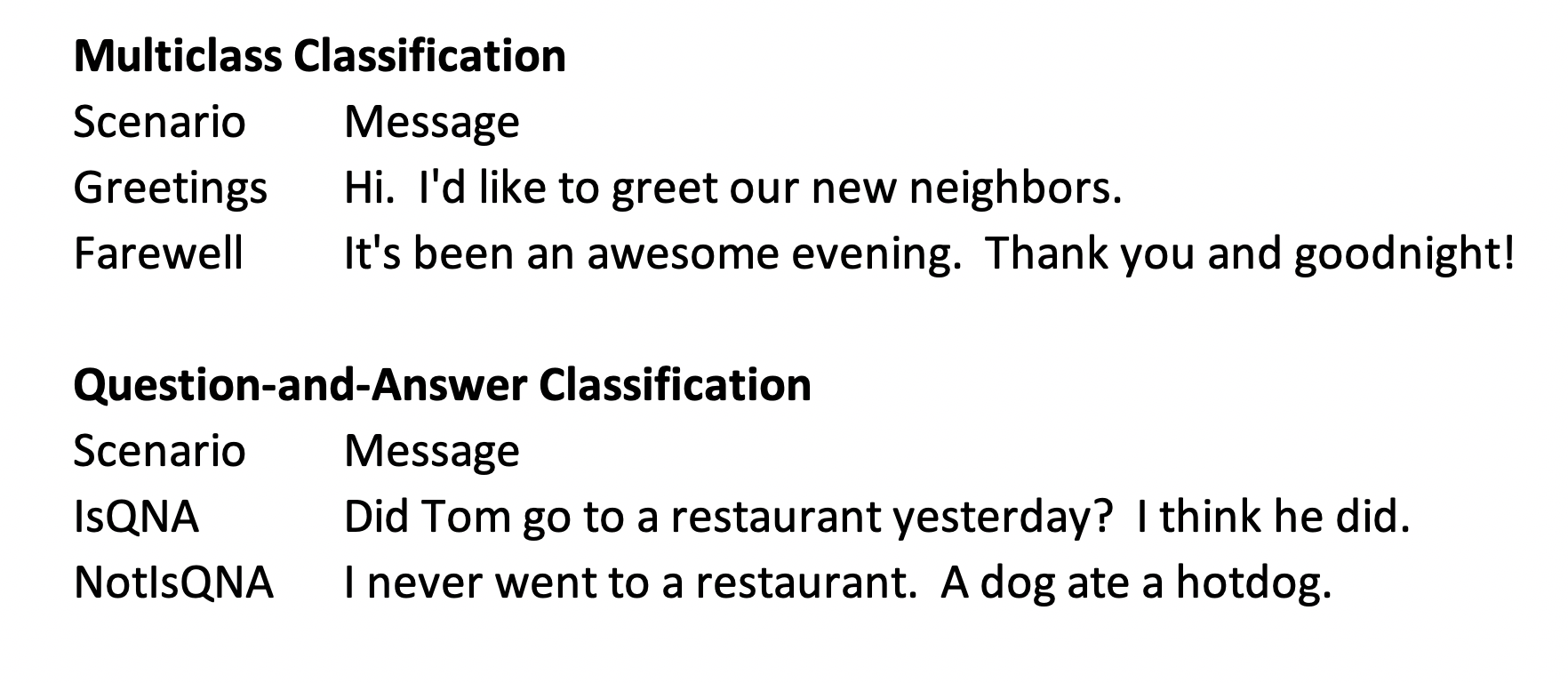# Step-by-Step BERT Explanation & Implementation Part 2— Data Formatting & Loading

Original article was published by Phillip Kim on Deep Learning on Medium# Step-by-Step BERT Explanation & Implementation Part 2— Data Formatting & Loading

This is a part 2 of the BERT Explanation & Implementation series. If you have not read Part 1 yet, it’s best to start from there. Let us continue from where we left off.

Data that I’m using have the following assumptions:

(1) The dataset contains multi-class labels, so we will be solving a multi-class classification problem. Label column is named “Scenario”.
(2) We will treat each message as a single sentence instead of multiple sentences. Thus each row in “Message” column will be treated as one sentence. The reason for this is that we are not solving a question-and-answer type of a problem. Rather, we want to solve a classification problem where we classify labels based on a message provided. We’d need to develop another parameter called token type ID to denote sentence separation in a given message to analyze the differences between one sentence to another if we were to solve a question-and-answer type of a problem. I will post another blog to specifically talk about this type of a problem in the future. A figure(a) below shows the difference.

`device = torch.device("cuda" if torch.cuda.is_available() else "cpu")torch.cuda.get_device_name(0)`

We set up a device here. If you have a GPU or GPUs, your device will be set to cuda if not cpu.

`category_to_id, id_to_category = bert_id_cat(df)labels = df['category_id'].tolist()sentences = df['Message'].tolist()sentences = bert_preprocess(sentences)tokenizer = BertTokenizer.from_pretrained('bert-base-uncased', do_lower_case=True)tokenized_texts = tokenizedTexts(tokenizer, sentences)`

We use pre-defined functions to preprocess our data. In addition, we load a pre-trained bert tokenizer model for sentence tokenization. You can download all 24 pre-trained bert models from here.

`input_ids = [tokenizer.convert_tokens_to_ids(x) for x in tokenized_texts]input_ids = pad_sequences(input_ids, maxlen=MAX_LEN, dtype="long", truncating="post", padding="post")`

Now we map tokens to input ids. We apply a pad sequence function to limit and fill all input ids to the length of 128. In Part 1, we set MAX_LEN = 128 for this reason. Truncation limits the number of tokens and Padding puts 0 in empty spaces so that the size is consistently 128. These functions make the size of each datum uniform throughout the entire dataset. Post truncation and padding means limiting the size from start to 128th place and putting 0’s in empty spaces that follow. Figure(b) and Figure(c) show examples of input ids and these functions.

`attention_masks = []for seq in input_ids:   seq_mask = [float(i>0) for i in seq]   attention_masks.append(seq_mask)`

We create attention masks by assigning the value of 0 for an input id with 0 and value of 1 otherwise. This tells the model that 0’s are padding and 1’s are actual tokens. So it knows which token to correctly mask for front and back bidirectional contextual prediction. This process can be seen somewhat redundant but it’s better to see 0’s and 1’s rather than 0’s and all possible numbers.

Now, we are ready to train BERT model with our prepared data.

`_, validation_data, _, validation_labelss = train_test_split(sentences, labels, random_state=2020, test_size=0.15)        train_inputs, validation_inputs, train_labels, validation_labels = train_test_split(input_ids, labels, random_state=2020, test_size=0.15)train_masks, validation_masks, _, _ = train_test_split(attention_masks, input_ids, random_state=2020, test_size=0.15)`

We split the entire dataset into training and test datasets using 85/15 ratio. We use random_state to make sure that all input data are correctly ordered. In the first lines of code, we only retrieve validation data and labels because we want to visually confirm our results later.

`train_inputs = torch.tensor(train_inputs)validation_inputs = torch.tensor(validation_inputs)train_labels = torch.tensor(train_labels)validation_labels = torch.tensor(validation_labels)train_masks = torch.tensor(train_masks)validation_masks = torch.tensor(validation_masks)`

We convert each input data format from list to torch tensor.

`batch_size = 32train_data = TensorDataset(train_inputs, train_masks, train_labels)train_sampler = RandomSampler(train_data)train_dataloader = DataLoader(train_data, sampler=train_sampler, batch_size=batch_size)validation_data = TensorDataset(validation_inputs, validation_masks, validation_labels)validation_sampler = SequentialSampler(validation_data)validation_dataloader = DataLoader(validation_data, sampler=validation_sampler, batch_size=batch_size)model = BertForSequenceClassification.from_pretrained("bert-base-uncased", num_labels=len(Base_Names))model.cuda()`

We use a batch size of 32 but we could change this to 64 or bigger if you have a powerful GPU. We combine relevant tensors into a tensor dataset. We want our training dataset to be randomly sampled but not test dataset. Hence the reason for random sampler for the training dataset and sequential sampler for the test dataset. Once the preparation is done, we load both tensor datasets into dataloaders. We use a pre-trained model for sequence classification. Finally, we set the model to use GPU for calculation. Now we are ready for fine-tuning of the model.

In Part 3, we will fine-tune parameters for the BERT model and train & test it. We will continue in Part 3 of the series.